# BPR 贝叶斯个性化排序

BPR是基于用户的隐式反馈，为用户提供物品的推荐，并且是直接对排序进行优化。

# 定义

$U$代表所有的用户user集合；

$I$代表所有的物品item集合；

$S$代表所有用户的隐式反馈，$S \subseteq U \times I$. 如下图所示，只要用户对某个物品产生过行为，就标记为$+$, 所有$+$样本构成了$S$。那些未观察到的数据（即用户没有产生行为的数据）标记为$?$.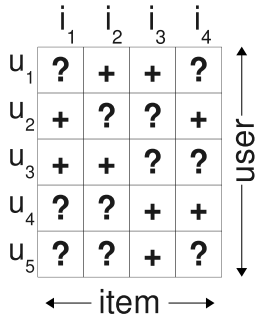$i>_uj$表示用户u在物品i和物品j之间更偏向于物品i

$I_u^+=\{i \in I: (u,i) \in S\}$ 代表了用户u产生过行为的物品集合

$U_i^+=\{u \in U: (u,i) \in S\}$ 代表了对物品i产生过行为的用户集合

# 传统解决方式# BPR的解决方式

BPR采用了pairwise的方式。

$D_S=\{(u,i,j)|i \in I_u^+ \land j \in I \backslash I_u^+\}$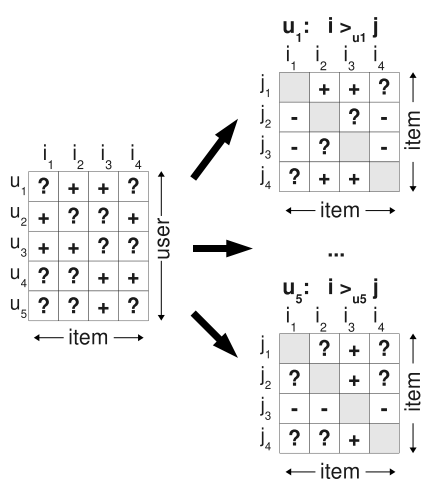# BPR-OPT

BPR基于最大后验概率, 对每一个用户u而言，后验概率正比于似然概率乘上先验概率：

$p(\theta|>_u) \propto p(>_u|\theta)p(\theta)$

## 似然概率

1. 所有用户之间的行为相互独立
2. 同一用户任意一对物品的偏序关系相互独立

$\Pi_{u\in U}p(>_u|\theta)=\Pi_{(u,i,j) \in D_S}p(i>_uj|\theta)$

$p(i>_uj|\theta):=\sigma(\hat{x}_{uij}(\theta))$

$\hat{x}_{uij}(\theta)$是个实值函数，返回的是用户u, 物品i, 物品j之间的关系。这个函数可以通过矩阵分解或者KNN等方法实现。

## 先验概率

$p(\theta) \sim N(0, \lambda_\theta I)$

$lnp(\theta)=\lambda||\theta||^2$

## 后验概率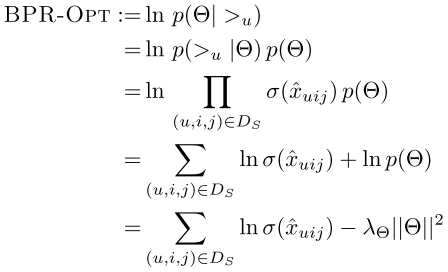## 梯度下降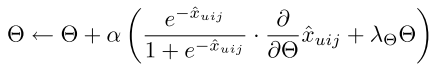# 基于矩阵分解的BPR

$\hat{X}=WH^T$

$\hat{x}_{ui}=w_u \cdot h_i=\sum_{f=1}^kw_{uf}h_{if}$

$\hat{x}_{ui}$的物理意义可以理解为是预测出的用户u对物品i的评分。

$\hat{x}_{uij}=\hat{x}_{ui}-\hat{x}_{uj}$

$lnp(\theta|>_u)=\sum_{(u,i,j)\in D_S}ln\sigma(\hat{x}_{ui}-\hat{x}_{uj})+\lambda||\theta||^2$

$\theta \leftarrow \theta + \alpha(\frac{1}{1+e^{\hat{x}_{ui}-\hat{x}_{uj}}} \cdot \frac{\partial}{\partial \theta}(\hat{x}_{ui}-\hat{x}_{uj})+\lambda\theta)$

$\hat{x}_{ui}-\hat{x}_{uj}=\sum_{f=1}^kw_{uf}h_{if}-\sum_{f=1}^kw_{uf}h_{jf}$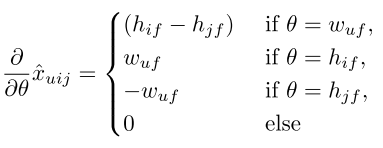Rendle, et.al., BPR: Bayesian Personalized Ranking from Implicit Feedback©️2019 CSDN 皮肤主题: 大白 设计师: CSDN官方博客